# Quiz 14: Cost Planning

Business

Calculate the number of units to be produced in June and July: Multiply the number of goods to be produced in the current month and it percentage and number of units to be produced in the following month and it percentage. Add the two percentages calculations to arrive at number of units to be produced.Number of units to be produced in June is:. Therefore, number of units produced in June is 13,200. Number of units to be produced in July is:Therefore, number of units produced in July is 15,400. Working note: Calculate the production units for the month of June:Calculate the production units for the month of July.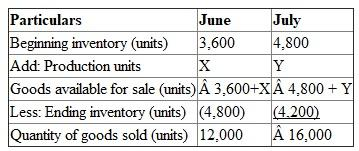Calculate the number of pounds of raw material to be purchased in June: To calculate units of pounds of raw material, multiply with material needed per unit and add the desired units of material in the ending inventory and subtract units of material in the beginning inventory.Therefore, the number of pounds of raw material to be purchased in June is 41,250 pounds.

According to the provided information, the total variable expenses are: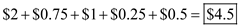The total fixed expenses are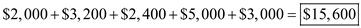The total variable expense for June is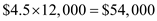. The total variable expense for July is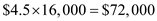. The total variable expense for August is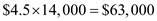. The total selling expenses for June are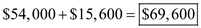. The total selling expenses for July are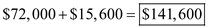. The total selling expenses for August are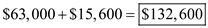. Therefore, the company's operating expense budget for June, July, and August is as below: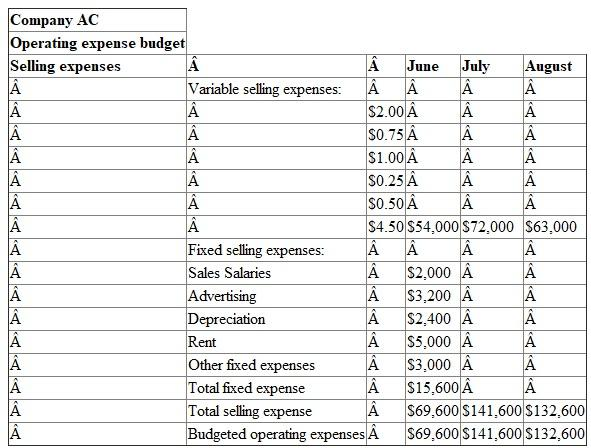There is no answer for this question

There is no answer for this question